## An R Companion for the Handbook of Biological Statistics

Salvatore S. Mangiafico

# Curvilinear Regression

When to use it

Null hypotheses

Assumptions

How the test works

Examples

Graphing the results

Similar tests

See the Handbook for information on these topics.

### How to do the test

This chapter will fit models to curvilinear data using three methods:  1) Polynomial regression;  2) B-spline regression with polynomial splines;  and 3) Nonlinear regression with the nls function.  In this example, each of these three will find essentially the same best-fit curve with very similar p-values and R-squared values.

#### Polynomial regression

Polynomial regression is really just a special case of multiple regression, which is covered in the Multiple regression chapter.  In this example we will fit a few models, as the Handbook does, and then compare the models with the extra sum of squares test, the Akaike information criterion (AIC), and the adjusted R-squared as model fit criteria.

For a linear model (lm), the adjusted R-squared is included with the output of the summary(model) statement.  The AIC is produced with its own function call, AIC(model).  The extra sum of squares test is conducted with the anova function applied to two models.

For AIC, smaller is better.  For adjusted R-squared, larger is better.  A non-significant p-value for the extra sum of squares test comparing model a to model b indicates that the model with the extra terms does not significantly reduce the error sum of squares over the reduced model.  Which is to say, a non-significant p-value suggests the model with the additional terms is not better than the reduced model.

### --------------------------------------------------------------
### Polynomial regression, turtle carapace example
### pp. 220–221
### --------------------------------------------------------------

Input = ("
Length  Clutch
284      3
290      2
290      7
290      7
298     11
299     12
302     10
306      8
306      8
309      9
310     10
311     13
317      7
317      9
320      6
323     13
334      2
334      8
")

### Change Length from integer to numeric variable
###   otherwise, we will get an integer overflow error on big numbers

Data\$Length = as.numeric(Data\$Length)

### Create quadratic, cubic, quartic variables

library(dplyr)

Data =
mutate(Data,
Length2 = Length*Length,
Length3 = Length*Length*Length,
Length4 = Length*Length*Length*Length)

library(FSA)

Length Clutch Length2  Length3     Length4

1     284      3   80656 22906304  6505390336

2     290      2   84100 24389000  7072810000

3     290      7   84100 24389000  7072810000

16    323     13  104329 33698267 10884540241

17    334      2  111556 37259704 12444741136

18    334      8  111556 37259704 12444741136

##### Define the models to compare

model.1 = lm (Clutch ~ Length,                               data=Data)
model.2 = lm (Clutch ~ Length + Length2,                     data=Data)
model.3 = lm (Clutch ~ Length + Length2 + Length3,           data=Data)
model.4 = lm (Clutch ~ Length + Length2 + Length3 + Length4, data=Data)

##### Generate the model selection criteria statistics for these models

summary(model.1)

Coefficients:

Estimate Std. Error t value Pr(>|t|)

(Intercept)  -0.4353    17.3499   -0.03     0.98

Length        0.0276     0.0563    0.49     0.63

Multiple R-squared:  0.0148,  Adjusted R-squared:  -0.0468

F-statistic: 0.24 on 1 and 16 DF,  p-value: 0.631

AIC(model.1)

 99.133

summary(model.2)

Coefficients:

Estimate Std. Error t value Pr(>|t|)

(Intercept) -9.00e+02   2.70e+02   -3.33   0.0046 **

Length       5.86e+00   1.75e+00    3.35   0.0044 **

Length2     -9.42e-03   2.83e-03   -3.33   0.0045 **

Multiple R-squared:  0.434,   Adjusted R-squared:  0.358

F-statistic: 5.75 on 2 and 15 DF,  p-value: 0.014

AIC(model.2)

 91.16157

anova(model.1, model.2)

Analysis of Variance Table

Res.Df    RSS Df Sum of Sq      F  Pr(>F)

1     16 186.15

2     15 106.97  1    79.178 11.102 0.00455 **

###  Continue this process for the remainder of the models

 Model selection criteria for four polynomial models.  Model 2 has the lowest AIC, suggesting it is the best model from this list for these data.  Likewise model 2 shows the largest adjusted R-squared.  Finally, the extra SS test shows model 2 to be better than model 1, but that model 3 is not better than model 2.  All this evidence indicates selecting model 2. Model AIC Adjusted R-squared p-value for extra SS from previous model 1 99.1 - 0.047 2 91.2 0.36 0.0045 3 92.7 0.33 0.55 4 94.4 0.29 0.64

##### Compare models with compareLM and anova

This process can be automated somewhat by using my compareLM function and by passing multiple models to the anova function.  Any of AIC, AICc, or BIC can be minimized to select the best model.  If you have no preference, I might recommend using AICc.

model.1 = lm (Clutch ~ Length,                               data=Data)
model.2 = lm (Clutch ~ Length + Length2,                     data=Data)
model.3 = lm (Clutch ~ Length + Length2 + Length3,           data=Data)
model.4 = lm (Clutch ~ Length + Length2 + Length3 + Length4, data=Data)

library(rcompanion)

compareLM(model.1, model.2, model.3, model.4)

\$Fit.criteria

Rank Df.res   AIC   AICc    BIC R.squared Adj.R.sq p.value Shapiro.W Shapiro.p

1    2     16 99.13 100.80 101.80   0.01478  -0.0468 0.63080    0.9559    0.5253

2    3     15 91.16  94.24  94.72   0.43380   0.3583 0.01403    0.9605    0.6116

3    4     14 92.68  97.68  97.14   0.44860   0.3305 0.03496    0.9762    0.9025

4    5     13 94.37 102.00  99.71   0.45810   0.2914 0.07413    0.9797    0.9474

anova(model.1, model.2, model.3, model.4)

Res.Df    RSS Df Sum of Sq       F   Pr(>F)

1     16 186.15

2     15 106.97  1    79.178 10.0535 0.007372 **  ## Compares m.2 to m.1

3     14 104.18  1     2.797  0.3551 0.561448     ## Compares m.3 to m.2

4     13 102.38  1     1.792  0.2276 0.641254     ## Compares m.4 to m.3

##### Investigate the final model

model.final = lm (Clutch ~ Length + Length2,
data=Data)

summary(model.final)                  # Shows coefficients,
#  overall p-value for model, R-squared

Coefficients:

Estimate Std. Error t value Pr(>|t|)

(Intercept) -9.00e+02   2.70e+02   -3.33   0.0046 **

Length       5.86e+00   1.75e+00    3.35   0.0044 **

Length2     -9.42e-03   2.83e-03   -3.33   0.0045 **

Multiple R-squared:  0.434,   Adjusted R-squared:  0.358

F-statistic: 5.75 on 2 and 15 DF,  p-value: 0.014

library(car)

Anova(model.final, type="II")          # Shows p-values for individual terms

Anova Table (Type II tests)

Response: Clutch

Sum Sq Df F value Pr(>F)

Length      79.9  1    11.2 0.0044 **

Length2     79.2  1    11.1 0.0045 **

Residuals  107.0 15

##### Simple plot of model

plot(Clutch ~ Length,
data = Data,
pch=16,
xlab = "Carapace length",
ylab = "Clutch")

i = seq(min(Data\$Length), max(Data\$Length), len=100)       #  x-values for line
predy = predict(model.final,
data.frame(Length=i, Length2=i*i))         #  fitted values
lines(i, predy,                                            #  curve
lty=1, lwd=2, col="blue")                            #  style and color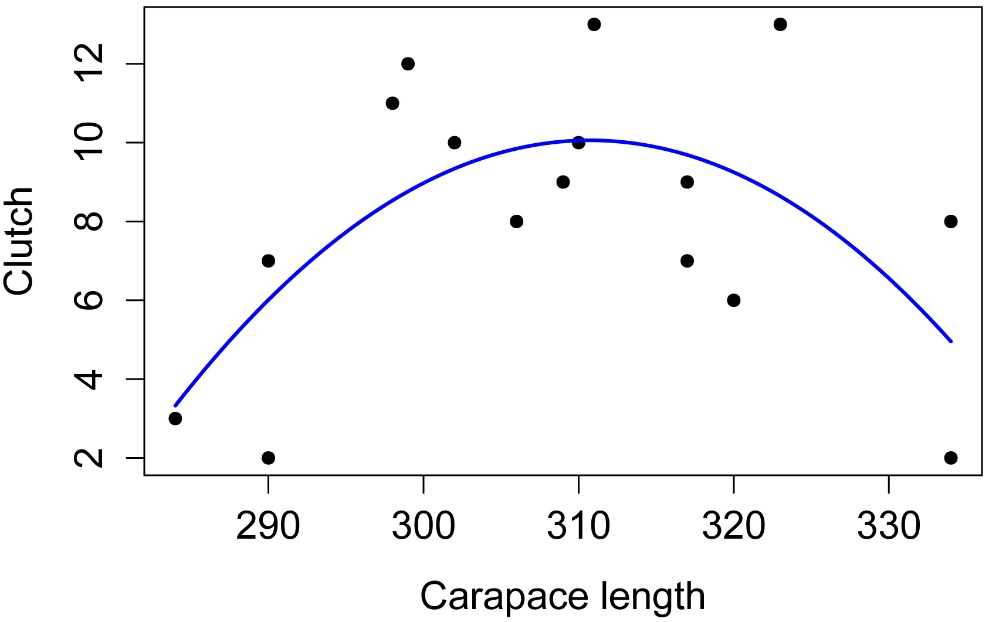##### Checking assumptions of the model

hist(residuals(model.final),
col="darkgray")A histogram of residuals from a linear model.  The distribution of these residuals should be approximately normal.

plot(fitted(model.final),
residuals(model.final))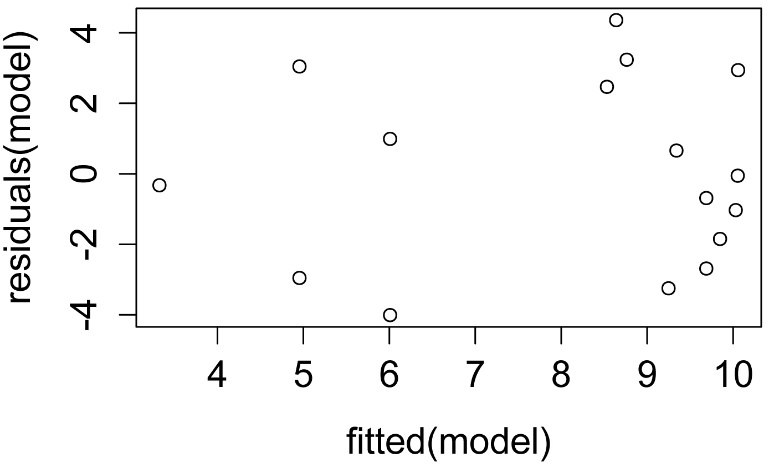A plot of residuals vs. predicted values.  The residuals should be unbiased and homoscedastic.  For an illustration of these properties, see this diagram by Steve Jost at DePaul University: condor.depaul.edu/sjost/it223/documents/resid-plots.gif.

### additional model checking plots with: plot(model.final)

#     #     #

#### B-spline regression with polynomial splines

B-spline regression uses smaller segments of linear or polynomial regression which are stitched together to make a single model.  It is useful to fit a curve to data when you don’t have a theoretical model to use (e.g. neither linear, nor polynomial, nor nonlinear).  It does not assume a linear relationship between the variables, but the residuals should still be normal and independent.  The model may be influenced by outliers.

### --------------------------------------------------------------
### B-spline regression, turtle carapace example
### pp. 220–221
### --------------------------------------------------------------

Input = ("
Length  Clutch
284      3
290      2
290      7
290      7
298     11
299     12
302     10
306      8
306      8
309      9
310     10
311     13
317      7
317      9
320      6
323     13
334      2
334      8
")

library(splines)

model = lm(Clutch ~ bs(Length,
knots = 5,     # How many internal segment nodes?
degree = 2),   # 1=local linear fits, 2=quadratic
data = Data)

summary(model)                         # Display p-value and R-squared

Residual standard error: 2.671 on 15 degrees of freedom

Multiple R-squared:  0.4338,  Adjusted R-squared:  0.3583

F-statistic: 5.747 on 2 and 15 DF,  p-value: 0.01403

##### Simple plot of model

plot(Clutch ~ Length,
data = Data,
pch=16,
xlab = "Carapace length",
ylab = "Clutch")

i = seq(min(Data\$Length), max(Data\$Length), len=100)       #  x-values for line
predy = predict(model, data.frame(Length=i))               #  fitted values
lines(i, predy,                                            #  spline curve
lty=1, lwd=2, col="blue")                            #  style and color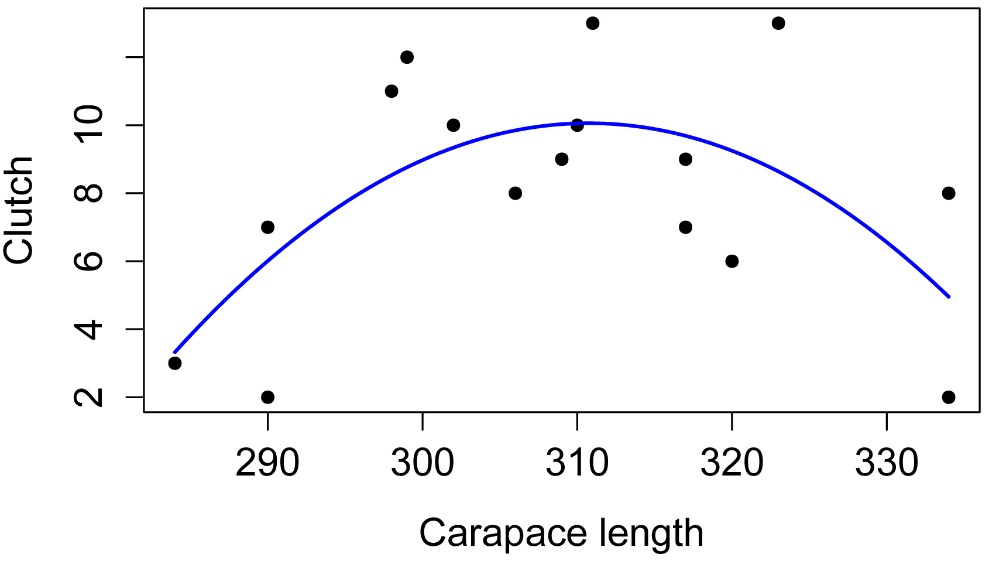##### Checking assumptions of the model

hist(residuals(model),
col="darkgray")A histogram of residuals from a linear model.  The distribution of these residuals should be approximately normal.

plot(fitted(model),
residuals(model))A plot of residuals vs. predicted values.  The residuals should be unbiased and homoscedastic.  For an illustration of these properties, see this diagram by Steve Jost at DePaul University: condor.depaul.edu/sjost/it223/documents/resid-plots.gif.

### additional model checking plots with: plot(model)

#     #     #

#### Nonlinear regression

Nonlinear regression can fit various nonlinear models to a data set.  These model might include exponential models, logarithmic models, decay curves, or growth curves.  The nls function works by an iterative process, starting with user supplied estimates for the parameters in the model, and finding successively better parameter estimates until certain convergence criteria are met.

In this example, we assume that we want to fit a parabola to our data, but we’ll use the vertex form of the equation ( y = a·(xh) + k ).  This form is handy because the point (h, k) indicates the vertex of the parabola.

Note in the formula in the nls call below, that there are variables from our data (Clutch and Length), and parameters we want to estimate (Lcenter, Cmax, and a).

There’s no set process for choosing starting estimates for the parameters.  Often, the parameters will be meaningful.  For example, here, Lcenter is the x-coordinate of the vertex and Cmax is the y-coordinate of the vertex.  So we can guess at reasonable values for these.  The parameter a would be difficult to guess at, though we know it should be negative because the parabola opens downward.

Because nls uses an iterative process based on initial estimates of the parameters, it fails to find a solution if the estimates are too far off, or it may return a set of parameter estimates that don’t fit the data well.  It is important to plot the solution and make sure it is reasonable.  I have seen nls have difficulty with models that have more than three parameters.  The package nlmrt uses a different process for determining the iterations, and may be better to fit difficult models.

If you wish to have an overall p-value for the model and a pseudo-R-squared for the model, the model will need to be compared with a null model.  Technically for this to be valid, the null model must be nested within the fitted model.  That means that the null model is a special case of the fitted model.  In our example, if we were to force a to be zero, that would leave a model Clutch ~ constant, where constant would be a parameter that estimates the mean of the Clutch variable.  Many theoretical models do not have this property; that is, they don’t have a constant or linear term.  They are therefore considered nonlinear models.  In these cases, nls can still be used to fit the model, but the extra steps determining the model’s overall p-value and pseudo-R-squared are technically not valid.  In these cases, models could be compared with the Akaike information criterion (AIC).

The p-value for the model, relative to the null model, is determined with the extra SS (F) test (anova function) or likelihood ratio test (lrtest in the package lmtest).

There are various pseudo-R-squared values that have been developed for models without r-squared defined.  My function nagelkerke calculates the McFadden, the Cox and Snell, and the Nagelkereke pseudo-R-squared.  For nls models, a null model must be explicitly defined and passed to the function.  The Nagelkereke is a modification of the Cox and Snell so that it has a maximum of 1.  I find the Nagelkereke to usually be satisfactory for nls, lme, and gls models.  As a technical note, for gls and lme models, my function uses the likelihood for the model with ML fitting (REML = FALSE).

Pseudo-R-squared values are not directly comparable to multiple R-squared values, though in the examples in this chapter, the Nagelkereke is reasonably close to the multiple R-squared for the quadratic parabola model.

### --------------------------------------------------------------
### Nonlinear regression, turtle carapace example
### pp. 220–221
### --------------------------------------------------------------

Input = ("
Length  Clutch
284      3
290      2
290      7
290      7
298     11
299     12
302     10
306      8
306      8
309      9
310     10
311     13
317      7
317      9
320      6
323     13
334      2
334      8
")

model = nls(Clutch ~ a * (Length - Lcenter)^2 + Cmax,
data   = Data,
start  = c(Lcenter = 310,
Cmax  =    12,
a     =    -1),
trace = FALSE,
nls.control(maxiter = 1000)
)

summary(model)

Parameters:

Estimate Std. Error t value Pr(>|t|)

Lcenter 310.72865    2.37976  130.57  < 2e-16 ***

Cmax     10.05879    0.86359   11.65  6.5e-09 ***

a        -0.00942    0.00283   -3.33   0.0045 **

##### Determine overall p-value and pseudo-R-squared

model.null = nls(Clutch ~ I,
data   = Data,
start  = c(I = 8),
trace = FALSE)

anova(model, model.null)

Res.Df Res.Sum Sq Df  Sum Sq F value  Pr(>F)

1     15     106.97

2     17     188.94 -2 -81.971   5.747 0.01403 *

library(rcompanion)

nagelkerke(fit  = model,
null = model.null)

\$Pseudo.R.squared.for.model.vs.null

Pseudo.R.squared

Cox and Snell (ML)                   0.433836

Nagelkerke (Cragg and Uhler)         0.436269

##### Determine confidence intervals for parameters

library(nlstools)

confint2(model,
level = 0.95,
method = "asymptotic")

2.5 %        97.5 %
Lcenter 305.6563154 315.800988774
Cmax      8.2180886  11.899483768
a        -0.0154538  -0.003395949

Boot=nlsBoot(model)

summary(Boot)

------
Bootstrap statistics
Estimate  Std. error
Lcenter 311.07998936 2.872859816
Cmax     10.13306941 0.764154661
a        -0.00938236 0.002599385

------
Median of bootstrap estimates and percentile confidence intervals
Median         2.5%         97.5%
Lcenter 310.770796703 306.78718266 316.153528168
Cmax     10.157560932   8.58974408  11.583719723
a        -0.009402318  -0.01432593  -0.004265714

##### Simple plot of model

plot(Clutch ~ Length,
data = Data,
pch=16,
xlab = "Carapace length",
ylab = "Clutch")

i = seq(min(Data\$Length), max(Data\$Length), len=100)       #  x-values for line
predy = predict(model, data.frame(Length=i))               #  fitted values
lines(i, predy,                                            #  curve
lty=1, lwd=2, col="blue")                            #  style and color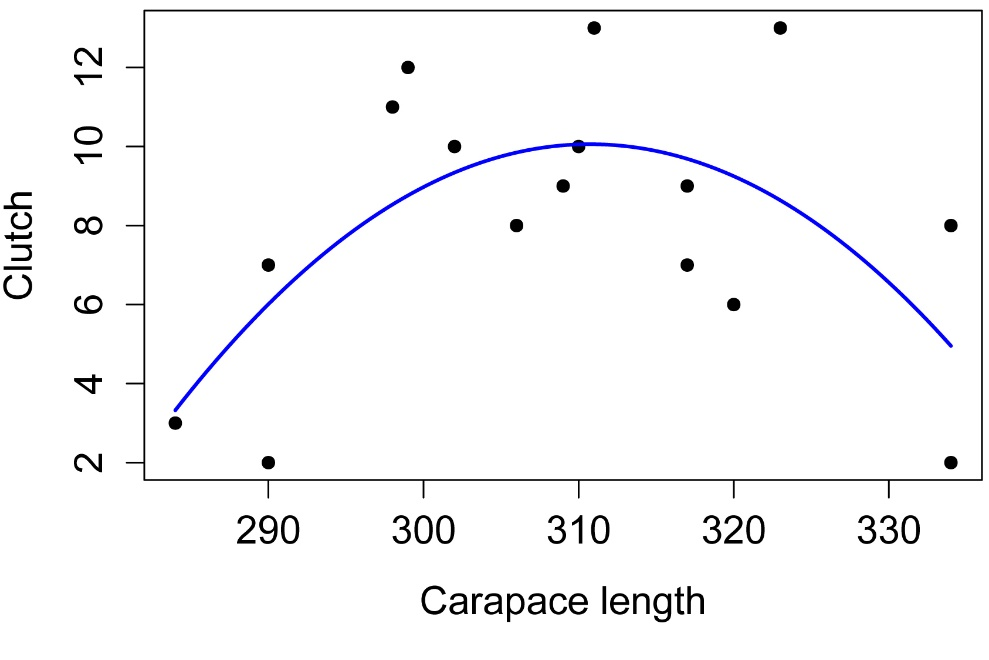##### Checking assumptions of the model

hist(residuals(model),
col="darkgray")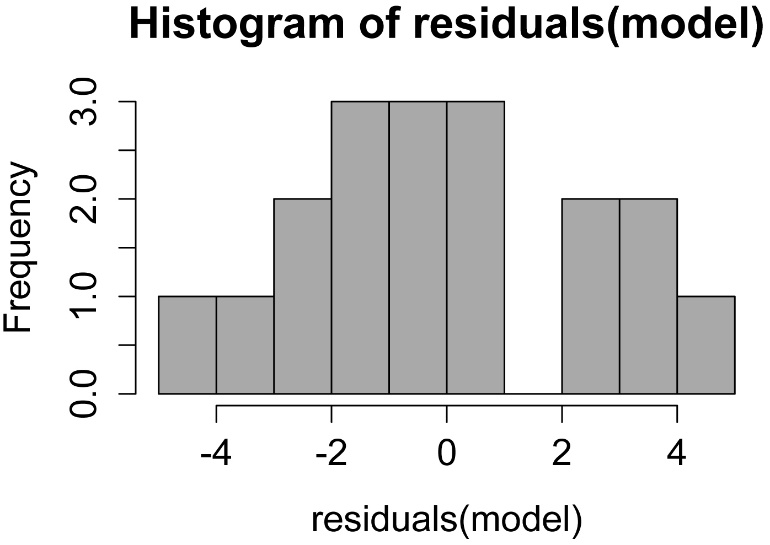A histogram of residuals from a linear model.  The distribution of these residuals should be approximately normal.

plot(fitted(model),
residuals(model))A plot of residuals vs. predicted values.  The residuals should be unbiased and homoscedastic.  For an illustration of these properties, see this diagram by Steve Jost at DePaul University: condor.depaul.edu/sjost/it223/documents/resid-plots.gif.

#     #     #